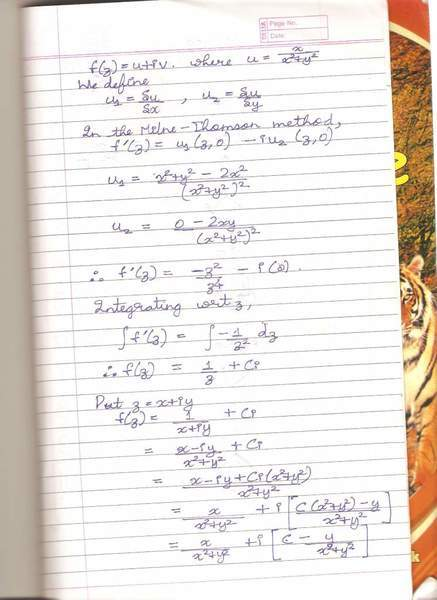# Finding the complex function given a real component

## Homework Statement

Find $$f(z) = u(x,y) + iv(x,y)$$ with u or v as a given.

## Homework Equations

$$u = \frac{x}{x^2+y^2}$$

## The Attempt at a Solution

Using the Cauchy-Riemann equations, if the function is analytic, then $$u_x = v_y$$ and $$u_y = -v_x$$

So, the first thing I did was find the x derivative of u.

$$u_x = \frac{y^2-x^2}{(x^2+y^2)^2}$$ which equals $$v_y$$

Then, I integrated in terms of y to get $$\frac{1}{x}\arctan{\frac{y}{x}} + A(x)$$

However, it seems that I might be going about this the wrong way, as the problem is getting rather hairy from here. The answer the book provides is much simpler than where this seems to be going.

HallsofIvy
Homework Helper
That's the right approach but I think you may have integrated incorrectly.

Dick
Homework Helper
It might be more obvious how to integrate the other Cauchy-Riemann equation, u_y=(-v_x). Try that.

I approached the problem from the other direction, and I am still ending up at an impasse. I am not entirely sure what to do with the A(y) function that results from the integration.

I'm getting:

$$v = \frac{y}{x^2+y^2} + A(y)$$

I am also getting
$$u_x = \frac{1}{x^2+y^2} - \frac{2x^2}{(x^2+y^2)^2}$$

Integrating with respect to y...
$$v = \frac{-y}{x^2+y^2} + B(x)$$

I get the following equality, after some rearranging:

$$B(x)-A(y) = \frac{2y}{x^2+y^2}$$

I am not entirely sure where to go from here, especially with regards to the A(x) and B(y), but the final complex function that the book was able to rearrange into was f(z) = 1/z.

So, I am still very much stuck as to how to continue.

Dick
Homework Helper
It looks to me like you got v=(-y)/(x^2+y^2). If so there's no need to continue, you are done, v and u satisfy the CR equations. And they are the real and complex parts of 1/z.

You could use the Milne-Thomson method to construct complex functions...Note : Pardon the handwriting in the image. C is the integration constant. Also, if you've been given the imaginary part of the complex function, you can use f ' (z) = v1(z , 0) + i v2(z , 0).

Last edited:
I should follow up on this. I still have the information regarding the Milne-Thomson method up, and am reading through it.

I realized that I had encountered an algebra mistake when I was doing the integration. I was able to figure it out, and I found out how the A(x) and B(y) functions disappeared just by comparing the two different forms of v.

Gib Z
Homework Helper
A good way to find the expression for the function in terms of $z$ is to use analytic continuation : The function on the real line must agree with the complex function. So just set y=0, and in the result replace all the x's with z's.